# Mathematics Quiz For RRB NTPC : 2nd January

Q1. 114×86= ?
(a) 8804
(b) 8814
(c) 8904
(d) 9804

Q2. Find the greatest number that will divide 43, 91 and 183 so as to leave the same remainder in each case.
(a) 4
(b) 7
(c) 9
(d) 13

Q3. यदि a का 20% = b, तो 20 का b% किस के बराबर होगा?
(a) 1.05
(b) 1.25
(c) 1.45
(d) 1.55

Q4.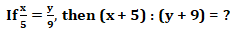(a) 4% of a
(b) 5% of a
(c) 20% of a
(d) None of these

Q5. A sum of Rs. 12,500 amounts to Rs. 15,500 in 4 years at the rate of simple interest. What is the rate of interest?
(a) 3%
(b) 4%
(c) 5%
(d) 6%

Q6. What will be the compound interest on a sum of Rs. 25,000 after 3 years at the rate of 12 p.c.p.a.?
(a) Rs. 9000.30
(b) Rs. 9720
(c) Rs. 10123.20
(d) Rs. 10483.20

Q7. If a man were to sell his table for Rs. 750, he would lose 25%. To gain 25%, he should sell it for:
(a) Rs. 1000
(b) Rs. 2000
(c) Rs. 15000
(d) None of these

Q8.(a) 3 : 5
(b) 13 : 8
(c) 5 : 9
(d) 9 : 5

Q9. If a person walks at 14 km/hr instead of 10 km/hr, he would have walked 20 km more. The actual distance travelled by him is:
(a) 50 km
(b) 56 km
(c) 70 km
(d) 80 km

Q10.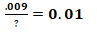(a) .0009
(b) .09
(c) .9
(d) 9

Solutions: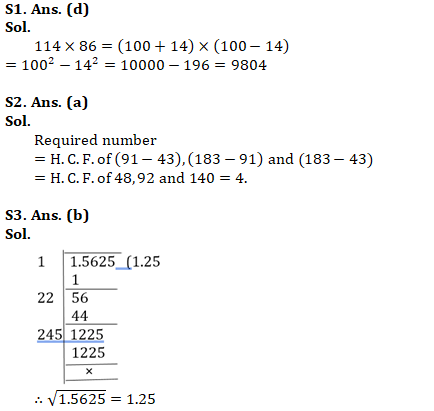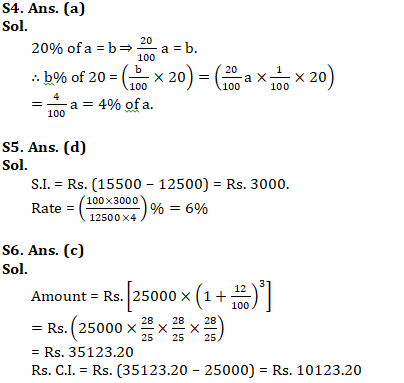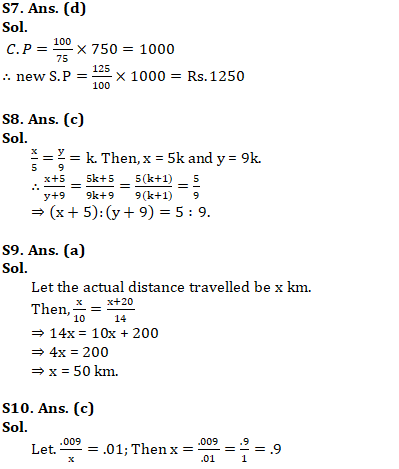Important Links for RRB NTPC Recruitment 2019